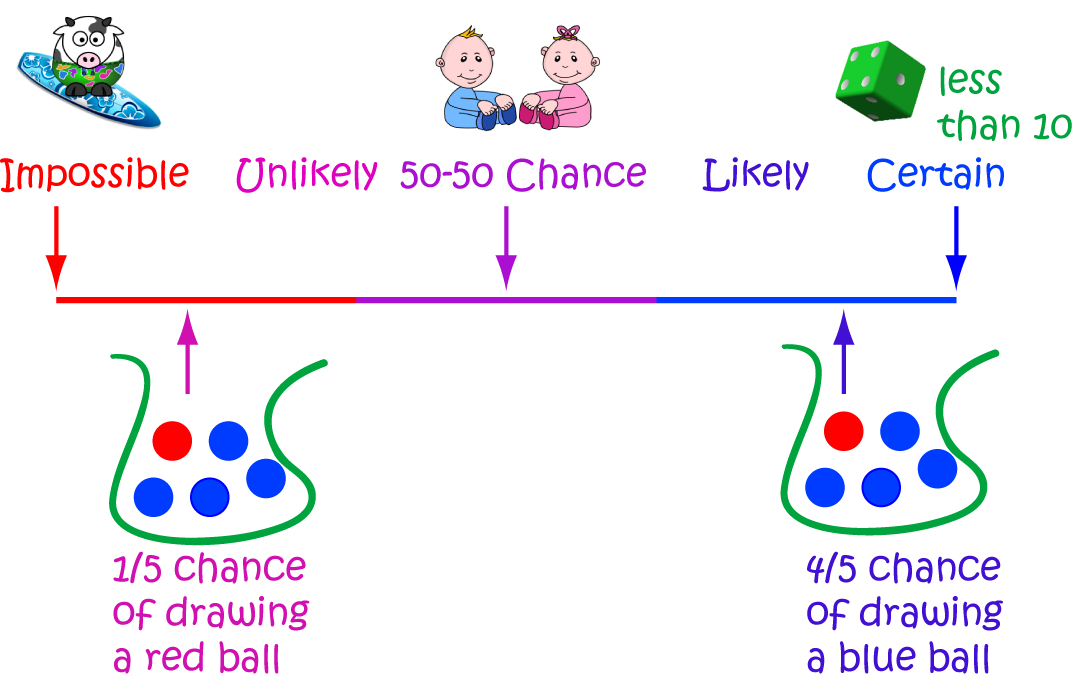# Definition of ProbabilityA probability tells you the chance that something will happen. In other words, it tells you how likely it is for a given event to happen.

Sometimes, probabilities are measured using percentages or fractions. For example, an event might be given a $25\%$ chance or a $\dfrac{1}{4}$ chance.

On other occasions, probabilities might be described in words like "unlikely", "possible", "50-50 chance", "likely" and "certain".

For example, the weather forecast might state that there's a $90\%$ chance of rain tomorrow. Then, it is likely to rain tomorrow.

If the weather forecast says there's a $100\%$ chance of rain tomorrow, it is certain that it will rain tomorrow.

### Description

The aim of this dictionary is to provide definitions to common mathematical terms. Students learn a new math skill every week at school, sometimes just before they start a new skill, if they want to look at what a specific term means, this is where this dictionary will become handy and a go-to guide for a student.

### Audience

Year 1 to Year 12 students

### Learning Objectives

Learn common math terms starting with letter P

Author: Subject Coach
You must be logged in as Student to ask a Question.